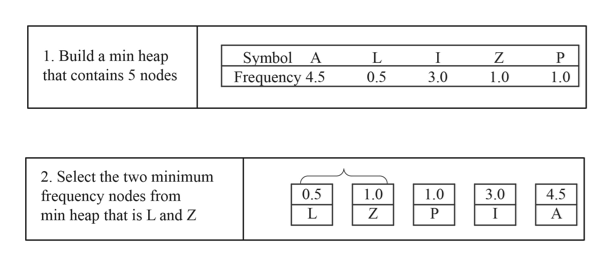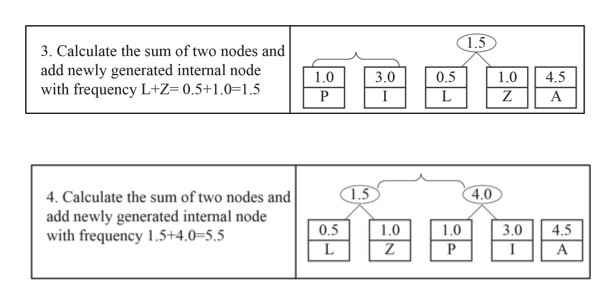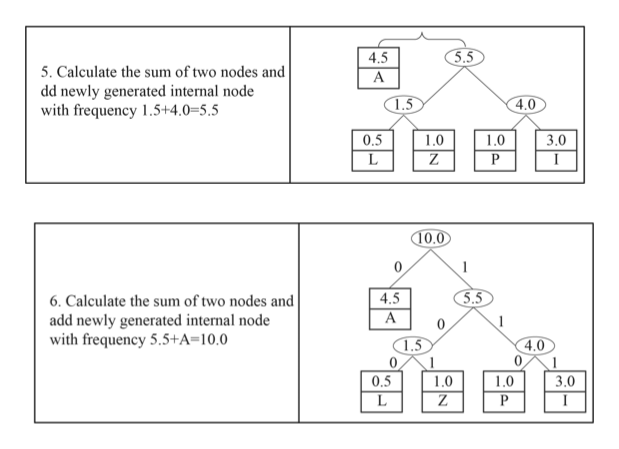# a. Construct a Huffman code for the following data:SymbolALIZPFrequency4.5.53.01.01.0b. Encode PLAZA using the code of part (a).c. Decode 10011101110110 using the code of part (a).

Question
105 views

a. Construct a Huffman code for the following data:

 Symbol A L I Z P Frequency 4.5 .5 3.0 1.0 1.0

b. Encode PLAZA using the code of part (a).

c. Decode 10011101110110 using the code of part (a).

check_circle

Step 1

a)

Constructing Hauffman code for the given data:help_outlineImage Transcriptionclose1. Build a min heap that contains 5 nodes Symbol A Frequency 4.5 Z P 0.5 3.0 1.0 1.0 2. Select the two minimum 0.5 3.0 1.0 1.0 4.5 frequency nodes from min heap that is L and Z P L Z I fullscreen
Step 2help_outlineImage Transcriptionclose1.5 3. Calculate the sum of two nodes and add newly generated internal node with frequency L+Z= 0.5+1.0=1.5 4.5 3.0 0.5 1.0 1.0 Z L 1.5 4.0 4. Calculate the sum of two nodes and add newly generated internal node with frequency 1.5+4.0=5.5 3.0 I 0.5 1.0 1.0 4.5 Z L P A fullscreen
Step 3help_outlineImage Transcriptionclose5.5 4.5 5. Calculate the sum of two nodes and dd newly generated internal node with frequency 1.5+4.0=5.5 A 4.0 1.5 1.0 Z 1.0 3.0 0.5 L P 10.0 4.5 5.5 6. Calculate the sum of two nodes and add newly generated internal node with frequency 5.5+A=10,0 A 1.5 4.0 0 0 0.5 3.0 1.0 10 L Z P fullscreen

### Want to see the full answer?

See Solution

#### Want to see this answer and more?

Solutions are written by subject experts who are available 24/7. Questions are typically answered within 1 hour.*

See Solution
*Response times may vary by subject and question.
Tagged in

### Computer Science